# The Shattering of SAT

Visualizing puddles and droplets

If condensed matter theorists have the Ising model, gravitational physicists have the Schwarzschild solution, and quantum foundation theorists have the Bell inequalities, then theoretical computer scientists have satisfiability, or SAT. In the world of computer science (and particularly computational complexity), many discussions inevitably circle back to SAT. In fact, SAT isn’t just something that theoretical computer scientists study. Satisfiability has a rich history with statistical physics, a field which wields powerful tools to probe the properties of SAT. As such, SAT is a problem which touches several fields, which makes it a breeding ground for cross-disciplinary ideas.

But what is satisfiability?

At its core, satisfiability is the search for optimizing constraints. When you’re trying to solve a problem, you often can’t give any sort of solution. You have to work within the limits of possibility, for starters. (This is why we have engineers instead of theoretical physicists building devices. The latter might get you something that technically works, but usually at the cost of neglecting air friction or imagining point masses.) You also have to take into account the wishes of the person giving you the problem. Roughly, the constraints you end up finding will be a combination of the laws of physics and the whims of the person.

Satisfiability is what you get when you abstract all the messiness of the real world and go to the land of computer science. We only care about the core idea, which is that you have variables for your system (think of possibilities for action) and constraints these variables must satisfy. From there, we can ask a variety of questions:

• Given a set of variables and constraints, can I set the variables such that they obey the constraints?
• How many solutions are there for a given set of constraints?
• If there is no solution, which configuration of the variables agrees with as many constraints as possible?

Each of these questions is a different way to tackle satisfiability. They called SAT, #SAT, and MAXSAT respectively1.

So what makes SAT such a magnet for research?

There are multiple reasons for this. First, most (but not all, see Reference 1) SAT problems belong to the complexity class NP, and are actually NP-complete. Without going into the details too much, because many SAT problems are NP-complete, it means that they are as difficult as any other problem in NP. If you have another NP problem and you suddenly find a way to solve a type of SAT in polynomial time, then all of the other problems in NP will also be solvable in polynomial time. As such, if you want to study the complexity class NP, you won’t do any better than studying SAT.

Second, SAT problems aren’t just of theoretical interest, but have a lot of practical applications.

One of those ideas from statistical physics that has been ported to SAT problems is the notion of phase transitions. At its core, a phase transition is the sudden radical change of a quantity that describes the system. The more radical the change, the “sharper” the transition. A classic example is in the Ising model, where temperature is the quantity you vary and the magnetization is the quantity that undergoes a sudden change (This is Figure 4 of these lecture notes).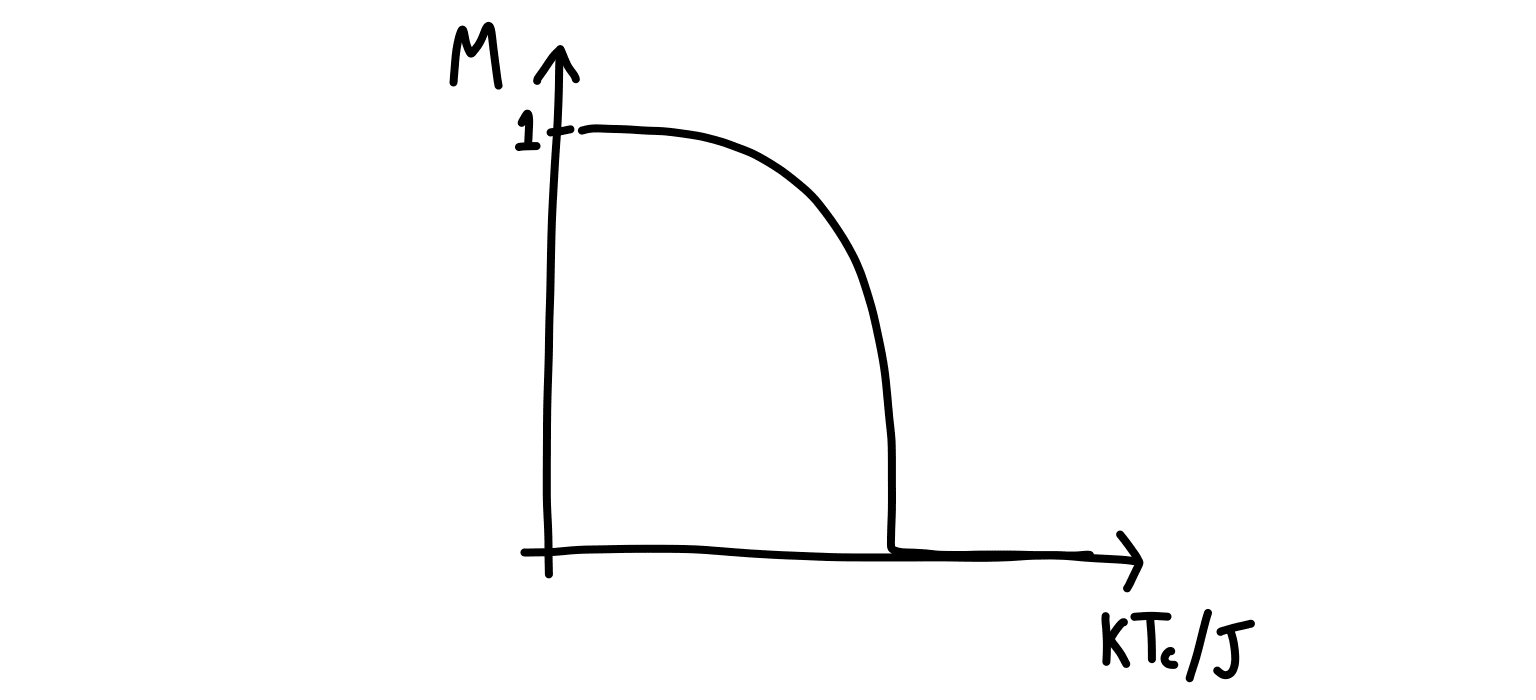Phase transitions indicate a drastic change in the system, and the toolkit of statistical physics is what lets us understand the way they work.

The reason I got hung up on phase transitions and SAT is because I came across the same diagram over and over again in SAT papers. Being physicists, they had the habit of making diagrams that only give you the idea of the concept instead of actually showing something from an experiment. In this case, the diagram looked something like this (see the References):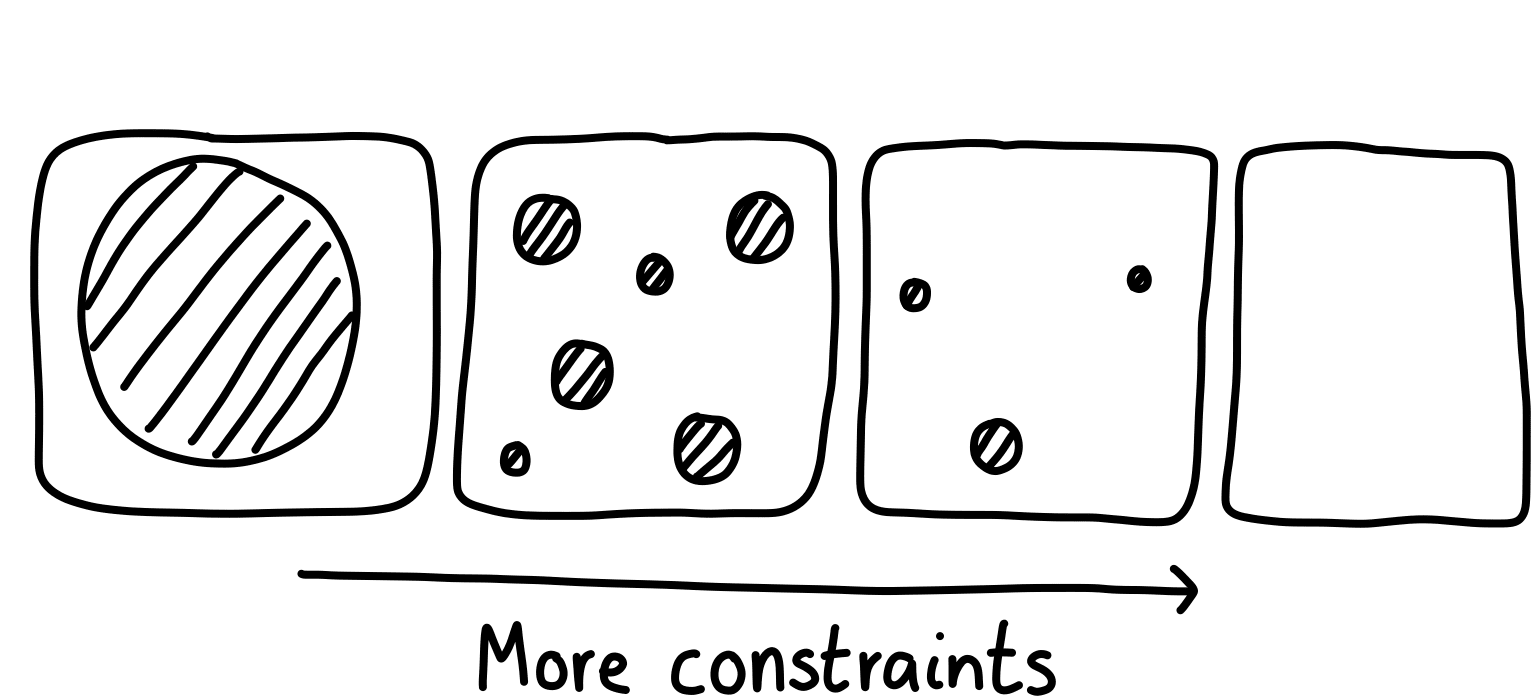If you read a few of these papers, you get the sense that the big blob on the left is the “puddle” of solutions when there aren’t many constraints, and as you add constraints, the puddle dries up and becomes just a collection of droplets. This is the “shattering” of SAT, and it means the solutions aren’t clustered close to each other (in terms of their bit strings).

That’s a fine mental model, but I wanted more. In particular, I wanted to understand what was going on to call the puddle one “thing”, while later on the puddle broke up into individual droplets. What was going on here?

In this essay, we’re going to look at some flavours of satisfiability, and understand where the critical thresholds for phase transitions are. The goal will be simple: To understand how the solution space of a SAT problem “shatters” or “clusters” as we increase the order parameter. To get there, we will have to venture into what a hypercube is, how to visualize SAT problems on graphs, and what it means to uncover phase transitions.

## Anatomy of a SAT problem

A SAT problem has a few parts. First, you have a set of variables. These variables can take values 0 or 1. From those variables, you have constraints. These constraints are in the form of tuples (think: lists) which specify what variables you have, and if they are negated.

For example, if I have V = 5 variables (x0, x1, x2, x3, x4), then a clause might be written as: (x0, x3, x4). This would tell me to include these three variables in a constraint. A SAT formula is just a bunch of constraints together.

The objective of a SAT problem varies depending on the question you ask, but if we take the first question I listed above, we simply want to know if there exists one way to set the variables so that all the constraints are satisfied. (In the jargon, the formula is a logical AND of all the constraints.)

As to the actual contents of each constraint, this is depends on the flavour of SAT you consider.

## Flavours of SAT

There are many different SAT variants, each with their own quirks. For this essay, I’m going to give you just a few variants so we can get a feel for what they are. For each variant, I’m going to use diagrams to give you an idea of how these constraints look. I’m also going to consider just k = 3 SAT problems here, though you could go higher (or lower to k = 2, but those end up being easier problems).

Before we move on, there’s an important point to make.

The two things we control for a given SAT variant is the number of variables V and the number of constraints C. It turns out that there are phase transitions when we α = C / V is a certain value (depending on the SAT variant). You can think of this as tell you how many constraints a given variable participates in one average. The idea for the phase transition is that below this threshold value of α, the probability that you will find at least one satisfying assignment is 1. Above the threshold, this probability drops to 0 (as you go to larger system sizes).

For us, we will see this a bit in the animations, but this threshold isn’t quite what we’re looking for in this essay. Instead, we want to see how quickly the solution space shatters, which by definition will have to happen before getting to the threshold (because then you have no solution). We won’t get into too deep the numerical values here. Instead, I just want to visualize what’s happening with these SAT variants.

### 3SAT

Plain old vanilla SAT is pretty simple. Everything’s a solution except for the all-zero configuration. If you have three variables, then (0,0,0) is the only solution that gets thrown out when you apply a clause.

As a diagram, it looks something like this: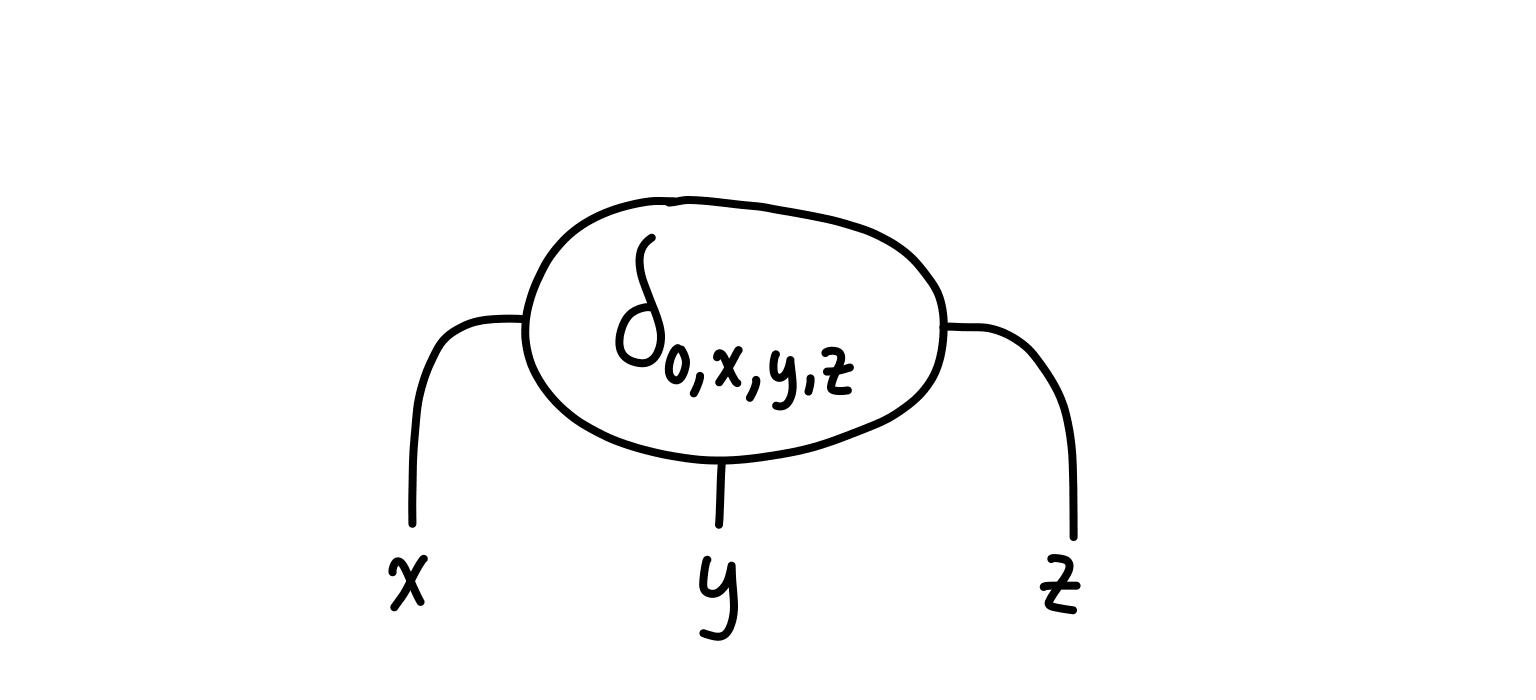Because a SAT clause only chucks out one of the eight possible assignments (in the case of three variables), you could imagine that you need a lot of clauses before there are no solutions left for your formula F. And you would be right! If we’re looking at 3SAT, then the threshold for satisfiability is at about α = C / V ~ 4.2 (see the References). I won’t show it in the animations below because of this high number of clauses needed to “break apart” the solution space, but I had to mention it.

### 3XORSAT

The “XOR” part of the name means “exclusive or”. It turns out that the XOR operation has a nice interpretation in terms of equations: Boolean addition, with a constraint added. This constraint is the parity of the clause, and can be even (0) or odd (1).

In other words, if we have three variables x, y, and z, then an even parity XOR clause would look like this: x ⊕ y ⊕ z = 0.

This means the only solutions to satisfy the clause over three bits would be:

• (0,0,0)
• (0,1,1)
• (1,0,1)
• (1,1,0)

Diagrammatically, we could represent it like this: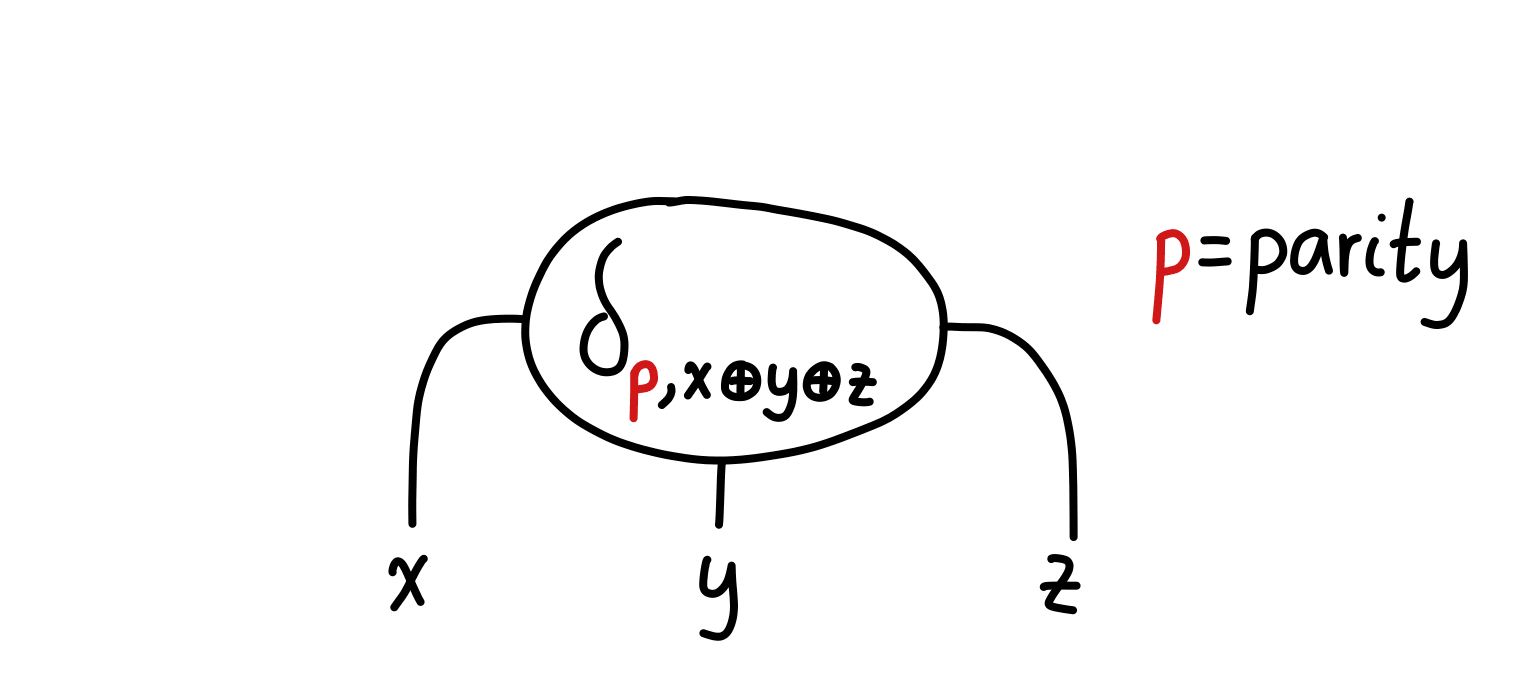There are two things to mention here. First, XORSAT is actually one of the examples of a SAT problem which is not in NP. It’s in P, and this has to do with the very special property that XORSAT can be recast as a series of Boolean equations. This can be solved by Gauss-Jordan elimination (that thing you did in linear algebra class a long time ago regarding row reductions and writing matrices out over and over). This is a polynomial time algorithm, so XORSAT is in P. But, XORSAT is still a problem that people study a lot2.

Second, the threshold for XORSAT (at least, a specific “constrained” version of it3) is at α = C / V = 1.

### 3NAESAT

The name for this one is “Not-All-Equal SAT”. I like to think of this as “double” the regular SAT problem. For regular SAT, you throw out the all-zeros configuration for the variables in a clause. For NAESAT, you throw out both the all-zeros and all-ones configurations.

As a diagram, it looks like this: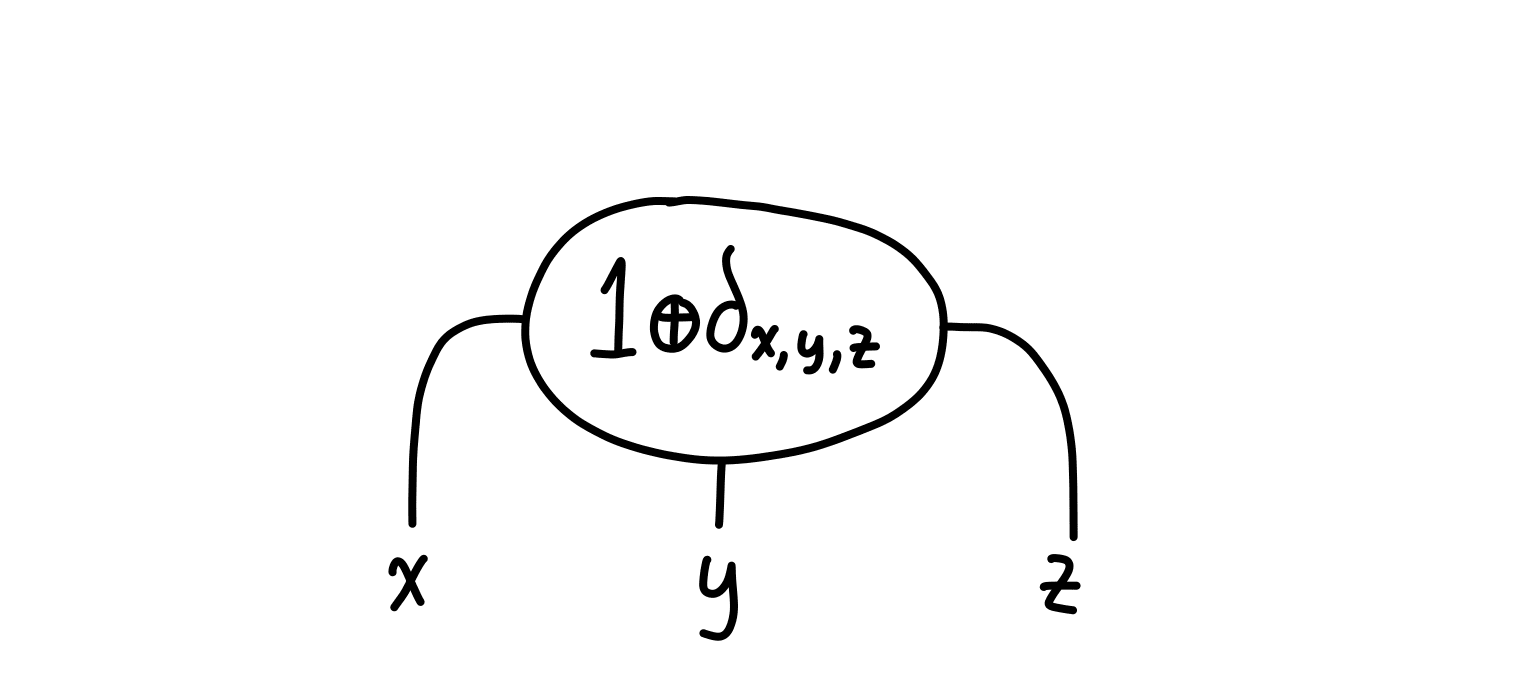In terms of the threshold, it’s located at α = C / V ~ 2.2.

### 1-in-3 SAT

Finally, we have 1-in-3 SAT. If we have three variables x, y, and z, this problem means the only accepted configurations are those that have exactly one 1 in their configuration. So the allowed configurations would be (1,0,0), (0,1,0), and (0,0,1).

As a diagram, it looks like this: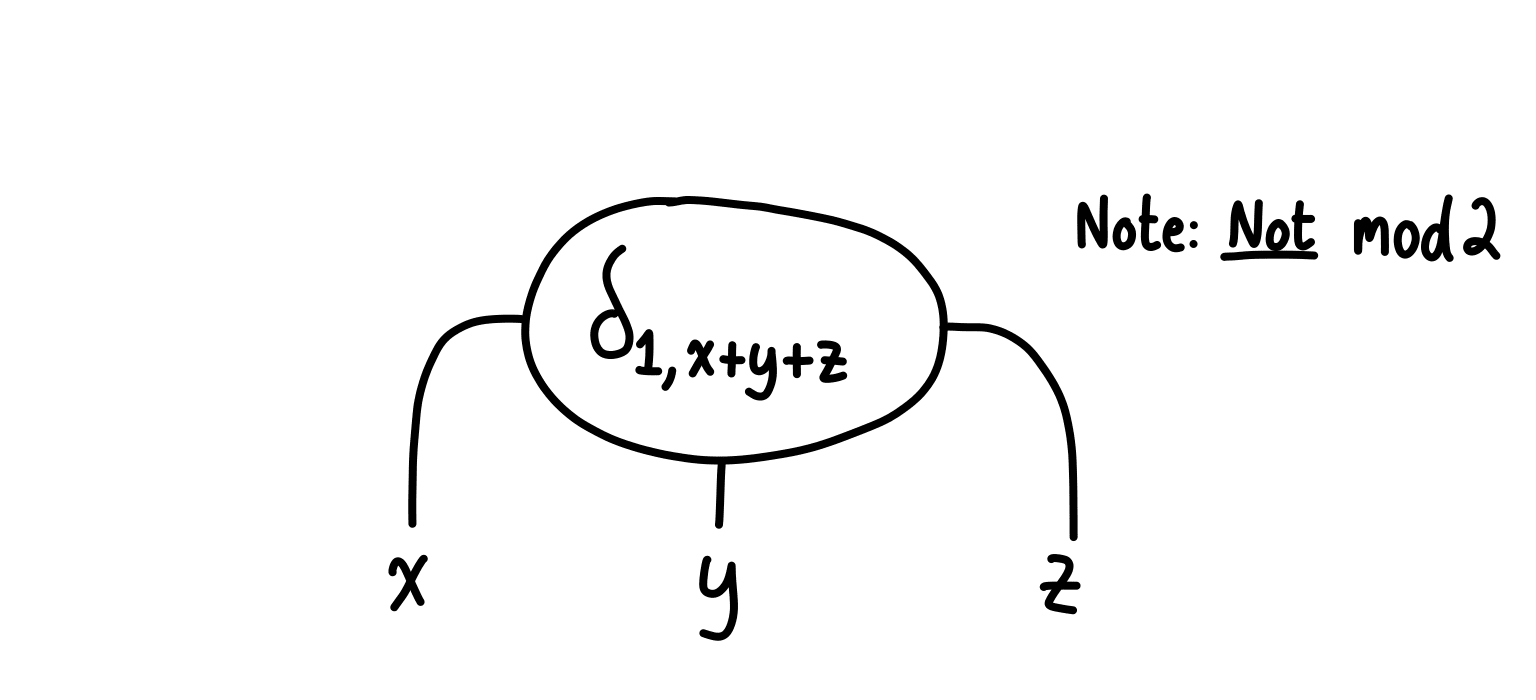Moreover, this variant is what you might call the most restrictive of all the variants. Whereas XORSAT filters out up to half the configurations each time (since we’re setting the parity, and it can be only even or odd), 1-in-3 SAT only allows 3/8 configurations. (For the general 1-in-k SAT problem, this becomes k / 2k configurations which are allowed.)

If you look at just positive 1-in-3SAT (see the next paragraph), the threshold is at α = C / V ~ 2/3.

This is just a quick look at the variants, since we’ll be seeing them in the experiments I‘ve run below. I should also add that we can also make things a bit more complicated by looking at clauses which have negated variables in them. Think of something like (~x, y, z), where the “~” symbol means NOT. This can happen, but for now, we’ll just focus our attention on the case where there are no negations. This is sometimes called “positive” SAT.

## SAT solutions on a hypercube

We’ve seen that different SAT variants have a threshold, so now let’s try to visualize how they evolve as we apply clauses.

To begin with, how do we visualize the set of solutions? There are many ways you can do this, but I want to take the one that is perhaps simplest and easy to think about.

If we have V variables, then there are precisely 2V configurations of bit strings. A nice way to see this is to think about building the bit string bit by bit. For the first bit, you have two choices: 1 or 0. For the second bit, you have two choices again: 1 or 0. Using the rule of multiplication, this means you have 2 × 2 = 22 = 4 choices. Continuing on for each variable gives the desired 2V.

We can represent these configurations in binary notation. If we have V = 3, then we get the following:

• 000
• 001
• 010
• 011
• 100
• 101
• 110
• 111

What’s really cool here is that this gives us a way to map bit strings to coordinates. If we imagine a 3D space (because we are dealing with 3 variables), then the bit strings can act as coordinates for our nodes.

That’s great, but if we are going to see the shattering of SAT, don’t the nodes have to cluster in some way?

Yes, here’s how.

We’re going to put an edge between nodes if we can “reach” one node by modifying the bit string of another in exactly one place.

Here’s an example. Say we start with the bit string 010. We have exactly three options for how to change it.

• Changing the first bit gives 010 &rightarrow; 110.
• Changing the second bit gives 010 &rightarrow; 000.
• Changing the third bit gives 010 &rightarrow; 011.

So we would connect the bit string 010 to the nodes at 110, 000, and 011.

What you will eventually find if you do this for the V = 3 case is something like this.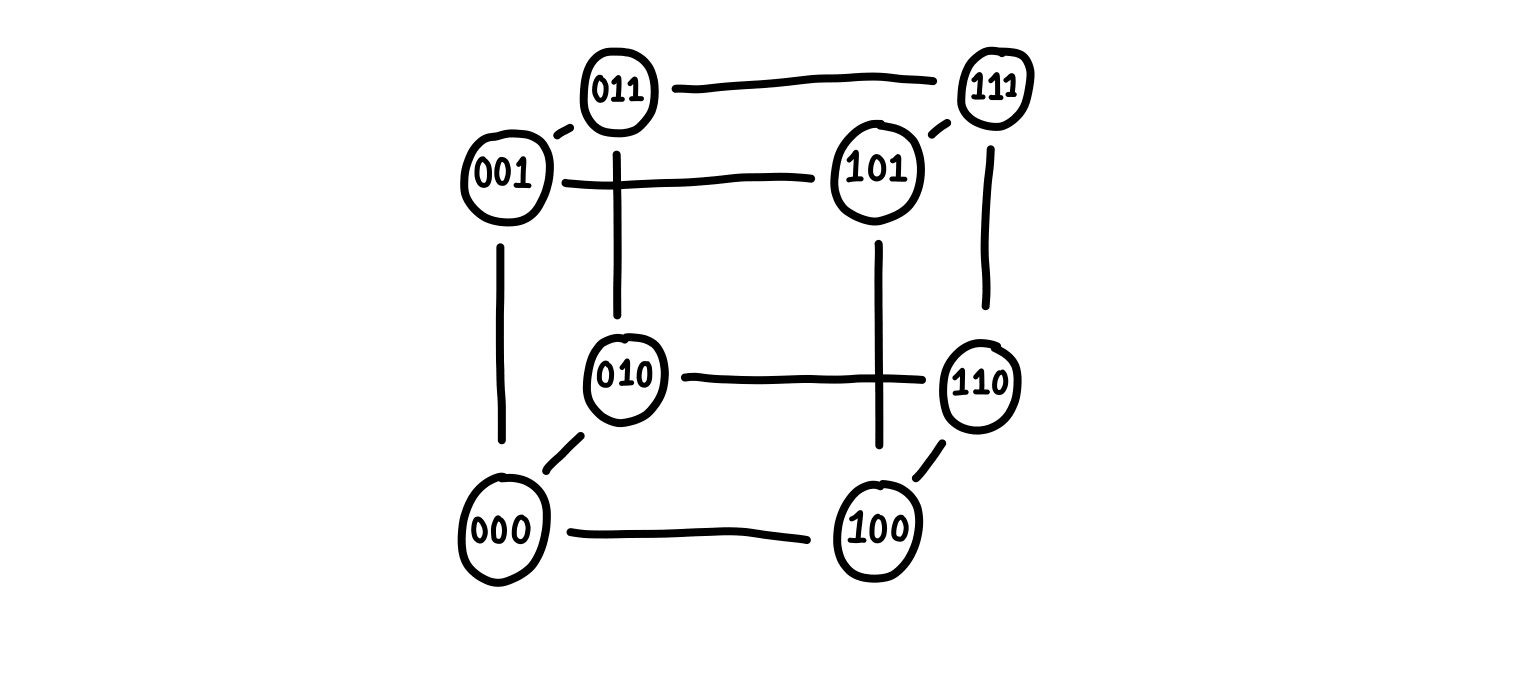Looks like a cube, doesn’t it?

In fact, that’s precisely what this structure is. When you flip one bit in a bit string, you’re connecting corners of what we call a hypercube, which is just the generalization of the cube into all dimensions greater than one.

This gives us a nice way of visualizing all of the potential SAT solutions: They are the corners of a hypercube.

Now, it’s important to note two things here. First, the graph that I’m showing here is different than the one we started from dealing with variables and constraints. This is a graph of the space of possible configurations for V variables. Nowhere here do we see anything about clauses. Instead, it’s just the solution space.

Second, the form of the graph is particular to our choice of connections. I told you that an edge means the bit strings are separated by one bit flip. This isn’t the only way to connect solutions. If I wanted, I could connect every node to any node that I can reach by two bit flips. That’s perfectly allowed.

So why am I limiting myself to one bit flip?

One reason is that the visualizations we’re going to see are done on a small number of variables. As such, if you connect solutions that are separated by multiple bit flips, your graph becomes really connected. And so you don’t end up seeing the shattering as effectively.

The other has to do with the premise of why these shattered spaces are of interest. Many algorithms for solving SAT problems are local, which means they branch out from one variable. The idea is that if the solution space gets shattered, it will be very difficult for a search algorithm which is in one cluster to branch out and “find” the other one, since they are separated by multiple bit flips. You could argue that two bit flips isn’t a lot, but for now, we’ll stick with one.

With this set up, the game becomes the following. Start with a hypercube in V dimensions. Label the nodes as bit strings corresponding to possible configurations for your SAT problem. Then, apply clauses. For each clause, figure out which configurations are incompatible with that clause, and remove those nodes from the graph (removing the nodes also removes the edges attached to them). At the end, if you still have nodes left in your graph, then those are precisely the solutions to your SAT problem.

To me, this is an elegant way of thinking about SAT problems. We’re pruning the hypercube of solutions, and seeing what’s left over.

## Seeing the droplets

For modern SAT solvers, you can go to quite a few variables (in the hundreds or thousands, I believe). In our case, this would be really bad when looking at the graph of solutions, since we would have to start with 2V nodes. Putting V = 1000 would be a really bad idea.

To keep things easy (and so I can take the shortcut of solving this through brute force), I’m going to limit our exploration to V = 12. This gives us just over four thousand solutions, which I think is reasonable for graphing.

The best way to implement this would be a SAT solver which can give you all solutions to a given SAT formula. In my case, I’m going to simply enumerate all the possible bit strings, and filter the list as I include more clauses. Then, I will plot the remaining nodes using the graphing package NetworkX. It’s as simple as that.

To generate the clauses, there are a few methods that can be used. Perhaps the easiest is the following: Using a random number generator, choose three random numbers (without replacement!) between 0 and V-1, and this tuple will become your clause. Repeat this for the number of clauses you want to use, and that’s it. If you want to be fancy, you can also include negations for the literals you choose with probability 1/2, but I’m not going to do that here. The only real difference is in 1-in-k SAT, where the threshold doubles if you avoid negations.

So that’s the setup. Let’s see some droplets!

For 3XORSAT: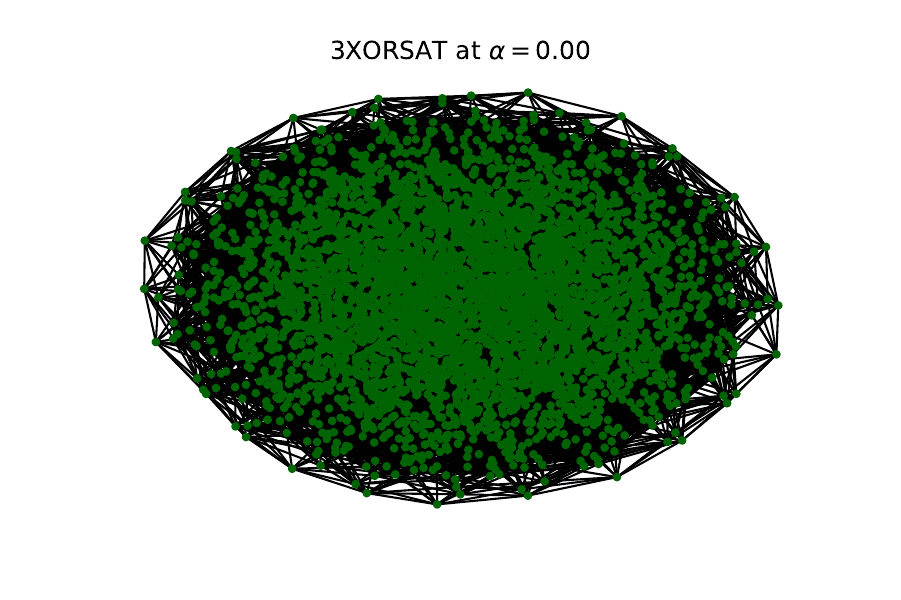For 3NAESAT: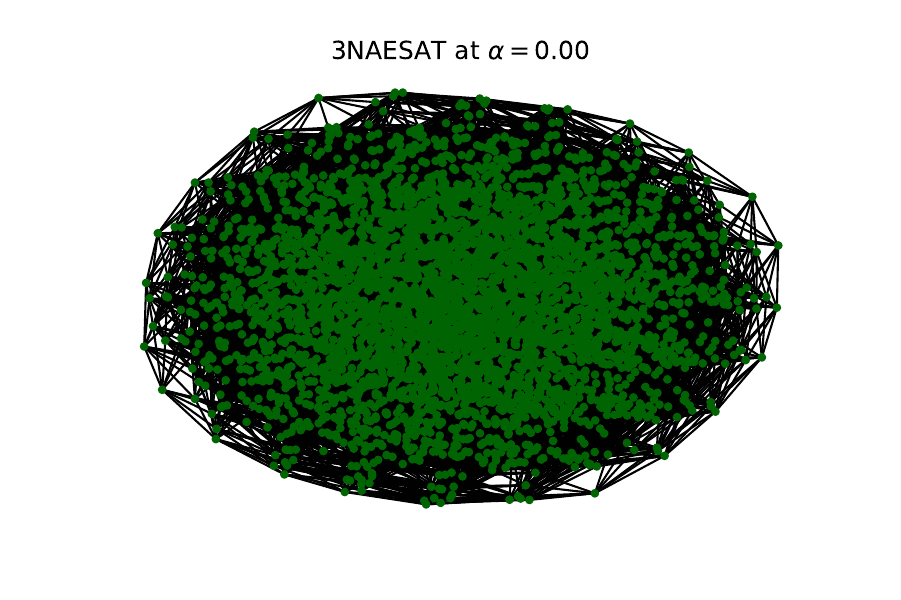For 1-in-3 SAT: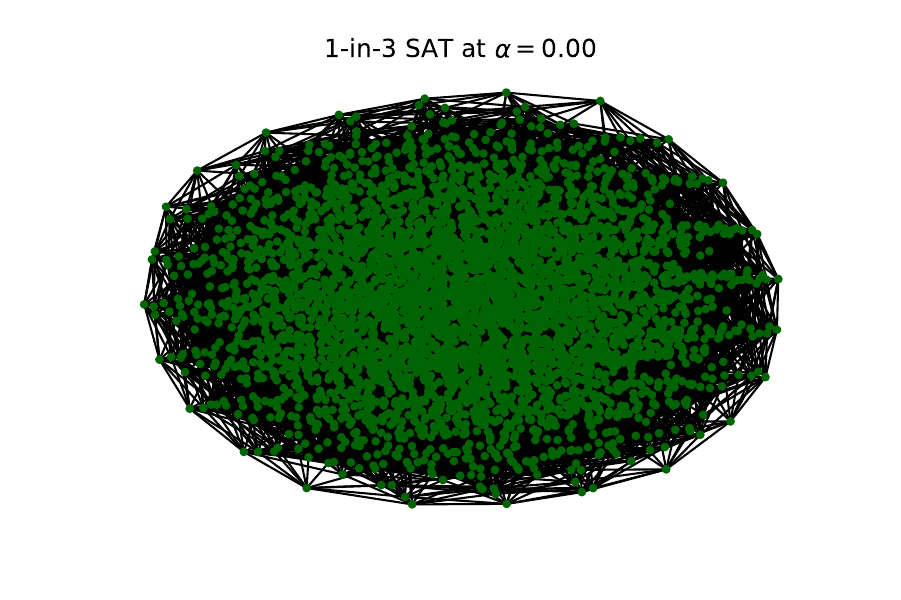I’m happy this visualization actually maps onto the simple diagram that I saw in all of those SAT papers and drew at the top of this essay. There are still many questions that could be asked about the exact locations of the thresholds for shattering, but that’s not the point of this essay. Instead, I wanted to give you a feel for what’s going on in the solution space.

One thing to note is that the visualization would change if we use a looser definition of when to draw edges (meaning a Hamming distance of greater than one).

The thing I find the most neat about all of this is how a very general and abstract computer science problem can worm its way into the minds of physicists and become relevant to them. To me, this signals that the toolkit of each scientist is useful, and you never know when it will be fruitfully applied to a new problem. The language of phase transitions isn’t just for the realm of materials and chemicals, but we can form strong analogies to more abstract problems.

And if that doesn’t satisfy you, I don’t know what will.

1. I’m not super familiar with this paper, but Schaefer’s dichotomy theorem seems to categorize the different types of SAT problems in terms of their complexity. See the Wikipedia page for Boolean satisfiability for more on this.
2. If you look at slide 15 of this presentation by Lenka Zdeborová, you can see the droplets in action. Figure 1 of “Hiding Quiet Solutions in Random Constraint Satisfaction Problems”, by Florent Krzakala and Lenka Zdeborová also has it. I’ve seen it floating around in other places too.

## Endnotes

1. SAT just refers to the usual satisfiability problem of answering the question, “Is there a configuration for the variables such that the constraints are satisfied?” #SAT doesn’t just ask for the existence of a solution, but the number of solutions. Finally, MAXSAT asks for the solutions that have the maximum number of satisfied constraints.

2. There are a few reasons for this, but from what I can tell, the main reason is that XORSAT is actually a difficult problem once you prohibit Gauss-Jordan elimination. It’s like we wield a magical sword that is capable of beating XORSAT because of some coincidences, so we ban it. Another reason is that XORSAT can be formulated as an Ising-like model with three-spin interactions. Concretely, if you take a Hamiltonian of the form H = -J ∑ijk sisjsk, with your spin variables taking values of ±1, then if you define si = (-1)xi, with these new xi variables being 0 or 1, your Hamiltonian reduces to H = -J ∑ijk (-1)xi + xj + xk = -J ∑ijk xi ⊕ xj ⊕ xk

3. This has to do with how many constraints each variable is connected to. See this paper by Boris Pittel and Gregory B. Sorkin (note that I haven’t read this paper closely, but the abstract contains the information I’m referring to).